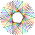CipherFeb 15, 2017

"Sudden death" of Kim Jong-un's half-brother by the numbers

http://edition.cnn.com/2017/02/14/asia/kim-jong-un-half-brother-death/index.html

This is a prime example of what they are talking about in the song "Murder By Numbers" by the Police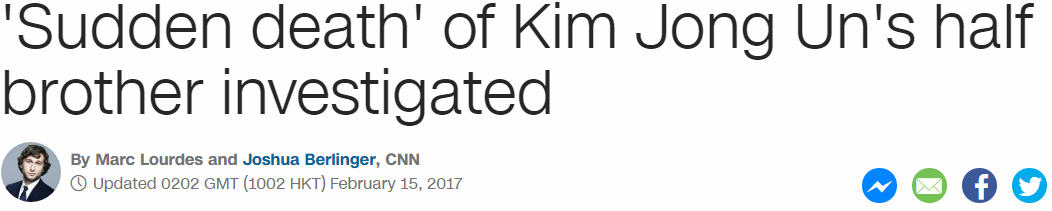This story is coming on 15th of February even though Kim Jong-nam died 2 days ago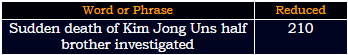Fifteenth of February = 6+9+6+20+5+5+14+20+8 + 15+6 + 6+5+2+18+21+1+18+25 = 210 (Ordinal) - Saturn = 21 R
Fifteenth of February = 6+9+6+100+5+5+40+100+8 + 50+6 + 6+5+2+80+200+1+80+400 = 1109 (Jewish) - Saturn = 119 F.B.Length of the video is 1:02 also connecting to the date of release
Fifteenth of February = 6+9+6+2+5+5+5+2+8 + 6+6 + 6+5+2+9+3+1+9+7 = 102 (Reduced)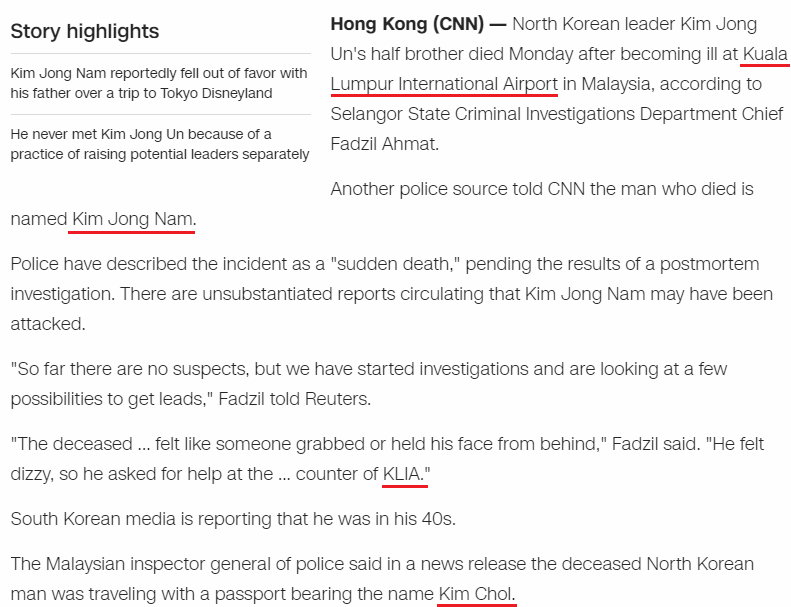Kim Jong-nam died on 13th of February which is the first day of LupercaliaLupercalia = 3+3+7+5+9+3+1+3+9+1 = 44 (Reduced)
Kim Jong nam = 2+9+4 + 1+6+5+7 + 5+1+4 = 44 (Reduced)
Kill = 44, Execution = 44..... and many more terms centered around death are connecting to 44
He "became ill" at "Koala Lumpur International Airport" while he was traveling with a fake passport under a name "Kim Chol"
Kuala Lumpur International Airport = 2+3+1+3+1 + 3+3+4+7+3+9 + 9+5+2+5+9+5+1+2+9+6+5+1+3 + 1+9+9+7+6+9+2 = 144 (Reduced)
Kim Chol = 11+9+13 + 3+8+15+12 = 71 (Ordinal)Kim Chol = 16+18+14 + 24+19+12+15 = 118 (Reverse Ordinal)
Death = 4+5+1+100+8 = 118 (Jewish)
His next birthday comes on 118th day after his death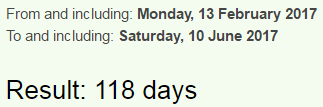Edit - I just went back to look at the post and noticed how they changed the video to be 1:18 longHe was caught using fake passport in the past and it definitely connects to this story (in this post they use the phrase "forged document" but when you go on Wikipedia they say "fake passport") - Kim_Jong-nam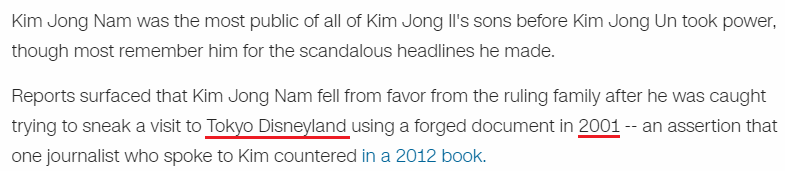Tokyo Disneyland has very relevant gematria connecting to a lot of 44 coding
Tokyo Disneyland = 20+15+11+25+15 + 4+9+19+14+5+25+12+1+14+4 = 193 (Ordinal)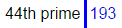Kim Jong-nam died 16 years after he tried to enter Tokyo Disneyland, on a date with 16 numerology while using fake passport again
2/13/2017 = 2+1+3+2+0+1+7 = 16Sixteen = 1+9+6+2+5+5+5 = 33 (Reduced), Kim = 11+9+13 = 33 (Ordinal)
Note that he is born on a date with 33 numerology ( 6 + 10 + 1 + 9 + 7 + 0 = 33), they say that he died after becoming "ill" = 9+12+12 = 33 (Ordinal)
fake passport = 6+1+2+5 + 7+1+10+10+7+6+9+2 = 66 (S Exception)
thirty three = 2+8+9+9+2+7 + 2+8+9+5+5 = 66 (Reduced)
"The deceased ... felt like someone grabbed or held his face from behind," Fadzil said. "He felt dizzy, so he asked for help at the ... counter of KLIA."
"KLIA" = 11+12+9+1 = 33 (Ordinal)
It's quite interesting how they split "The deceased" from the rest of the sentence
"felt like someone grabbed or held his face from behind" = 6+5+3+2 + 3+9+2+5 + 1+6+4+5+6+5+5 + 7+9+1+2+2+5+4 + 6+9 + 8+5+3+4 + 8+9+1 + 6+1+3+5 + 6+9+6+4 + 2+5+8+9+5+4 = 223 (Reduced)playboy = 7+3+1+7+2+6+7 = 33 (Reduced)
careless playboy = 24+26+9+22+15+22+8+8 + 11+15+26+2+25+12+2 = 227 (Reverse Ordinal)
"He spoke out against his father's 'military first' policy" = 8+5 + 1+7+6+2+5 + 6+3+2 + 1+7+1+9+5+1+2 + 8+9+1 + 6+1+2+8+5+9+1 + 4+9+3+9+2+1+9+7 + 6+9+9+1+2 + 7+6+3+9+3+7 = 227 (Reduced)Wikipedia -  "South Korean media reported that Kim was assassinated with poisoned needles by two unidentified women"
"assassinated" = 26+8+8+26+8+8+18+13+26+7+22+23 = 193 (Reverse Ordinal)
44th prime is 193 and this connects to Tokyo Disneyland
assassinated = 1+1+1+1+1+1+9+5+1+2+5+4 = 32 (Reduced) - 2+13+17 = 32
In the title CNN uses the phrase "Sudden Death"February = 6+5+2+9+3+1+9+7 = 42 (Reduced)
Kim Jong Un = 2+9+4 + 1+6+5+7 + 3+5 = 42 (Reduced)
Also ordinal sum corresponds to his birth date numerology
6/10/1970 = 6+10+19+70 = 105 (also it works with his edited date...5/10/1971 = 5+10+19+71= 105)

It's interesting how Kim Jong-nam dies a few days after North Korea's latest missile launch, and the first one in 2017
----------------------------------------------------------------------------------------------------------------------------------------------------------------------------------------------------------------------------------------------------
Ok... So I went back to the Wiki page of Kim Jong-nam 4 hours after releasing this post and guess what I see? https://en.wikipedia.org/wiki/Kim_Jong-nam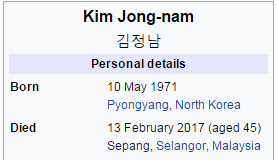His birthday is changed by entire year almost.... As I showed in the screenshot near the top of this post, Wikipedia had the birthday as 6/10/1970.... now they made it 5/10/1971, of course still a date with 33 numerology but it's very interesting measuring from his birthdays to the time of death, we have 227 and 93 coming upDied 2 months 27 days before his "next birthday".... I can't believe for a second that someone made such a ridiculous mistake like fucking up someone's birth dateNo comment.. but it's interesting how CNN never mentioned how old he was, they just said this - South Korean media is reporting that Kim was in his 40s.

1.2.